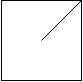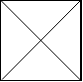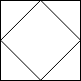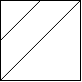| English | 简体中文 |

# 959. Regions Cut By Slashes

## Description

In a N x N grid composed of 1 x 1 squares, each 1 x 1 square consists of a /, \, or blank space.  These characters divide the square into contiguous regions.

(Note that backslash characters are escaped, so a \ is represented as "\\".)

Return the number of regions.

Example 1:

Input:
[
" /",
"/ "
]
Output: 2
Explanation: The 2x2 grid is as follows:Example 2:

Input:
[
" /",
"  "
]
Output: 1
Explanation: The 2x2 grid is as follows:Example 3:

Input:
[
"\\/",
"/\\"
]
Output: 4
Explanation: (Recall that because \ characters are escaped, "\\/" refers to \/, and "/\\" refers to /\.)
The 2x2 grid is as follows:Example 4:

Input:
[
"/\\",
"\\/"
]
Output: 5
Explanation: (Recall that because \ characters are escaped, "/\\" refers to /\, and "\\/" refers to \/.)
The 2x2 grid is as follows:Example 5:

Input:
[
"//",
"/ "
]
Output: 3
Explanation: The 2x2 grid is as follows:Note:

1. 1 <= grid.length == grid.length <= 30
2. grid[i][j] is either '/', '\', or ' '.